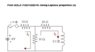# Laplace transform of the given circuit

• Engineering
Homework Statement:
Find i2(t) from given circuit
Relevant Equations:
Laplace transform of R-L circuit
Hello i have an assignment. From given circuit i need to find s domain and inverse them back to t domain.
can you help me by explain this circuit?

#### Attachments

•Form UTS_Matematika Teknik.jpg
11.4 KB · Views: 171

Staff Emeritus
Show us your work. We are here to help with hints or to correct if you did it wrong. We can't do that unless we see your attempt at the solution first.

im sorry,but i really have no idea. i really don't know what to do.
please show me some hints first and i will try it and post it here

Staff Emeritus
Our guidelines say this.
Finally, we want you to develop the learning skills you need to succeed in all of your classes. You have to be able to get past the "I have no clue" phase on your own.

So if you were assigned this as homework, then the instructor thinks that you should be ready to solve it. What have you studied in this course about Laplace transforms? Did the lectures show how to analyze a circuit using Laplace properties?

•JD_PM and berkeman
Mentor
what do you think? where i miss?
Please learn to type your work into the forum window. That makes it *much* easier to read and for us to quote it. There is a tutorial at the top of the page under INFO, Help.

You can also see how LaTeX cleans up the posting of math equations in this similar thread:

Homework Helper
Gold Member
As Berkeman suggested, try to type your work here.
If you insist on using pen and paper, re-draw the circuit, label the components and write the equations clearly, step by step.

Solution approach:
First, convert all the circuit components into their respective Laplace domain equivalents. Then you can go ahead with a circuit analysis method that you find convenient (node voltage method, mesh analysis, Thevenin/Norton etc).

•berkeman
from mesh analysis,is this formula corect?

Equation 1:
I1 RE + (I1-I2) R2 - Vs = 0
I1 (R1+R2) - I2 R2 = Vs
4 = I1 (R1+R2) - I2 R2
4 = I1 (10+R2) - I2 R2
I1 = (4 + 10 I2)/40

Equation 2:
-I1 R2 + I2 (R2+R3) + Ldi2/dt = 0

put equation 1 to 2
-10 I1 + I2 (10+20) + 0,1 di
2/dt=0
-((4 + 10 I2)/40) + 30 I2 + 0,1 Si2(s) - S i (0)=0
-4 - 10 I2/40 + 30 I2 + 0,1 S i2(s) = 0
-0,1 - 0,25 I2 + 30 I2 + 0,1 I2
(s) = 0
-0,1 + 29,75 I2 + 0,1 I2 (s) = 0
- 0,1 + I2 (29,75 + 0,1 (s)) = 0
I2 = 0,1 / 29,75 + 0,1 (s)
I2 = 0,1/s / 29,75/s + 0,1 (s)